Download Presentation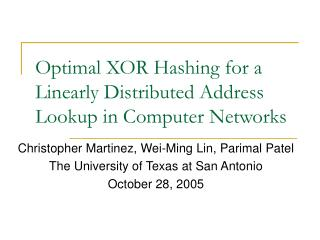Optimal XOR Hashing for a Linearly Distributed Address Lookup in Computer Networks

# Optimal XOR Hashing for a Linearly Distributed Address Lookup in Computer Networks - PowerPoint PPT Presentation

Optimal XOR Hashing for a Linearly Distributed Address Lookup in Computer Networks. Christopher Martinez, Wei-Ming Lin, Parimal Patel The University of Texas at San Antonio October 28, 2005. Outline. Motivation Hashing Background Linear Distribution Optimal Hashing Simulation Conclusion.I am the owner, or an agent authorized to act on behalf of the owner, of the copyrighted work described.
Download Presentation## Optimal XOR Hashing for a Linearly Distributed Address Lookup in Computer Networks

An Image/Link below is provided (as is) to download presentation

Download Policy: Content on the Website is provided to you AS IS for your information and personal use and may not be sold / licensed / shared on other websites without getting consent from its author.While downloading, if for some reason you are not able to download a presentation, the publisher may have deleted the file from their server.

- - - - - - - - - - - - - - - - - - - - - - - - - - E N D - - - - - - - - - - - - - - - - - - - - - - - - - -
Presentation Transcript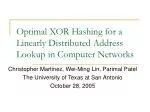### Optimal XOR Hashing for a Linearly Distributed Address Lookup in Computer Networks

Christopher Martinez, Wei-Ming Lin, Parimal Patel

The University of Texas at San Antonio

October 28, 2005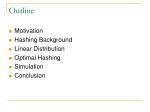Outline
• Motivation
• Hashing Background
• Linear Distribution
• Optimal Hashing
• Simulation
• Conclusion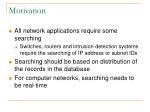Motivation
• All network applications require some searching
• Switches, routers and intrusion detection systems require the searching of IP address or subnet IDs
• Searching should be based on distribution of the records in the database
• For computer networks, searching needs to be real-time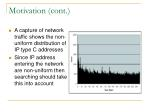Motivation (cont.)
• A capture of network traffic shows the non-uniform distribution of IP type C addresses
• Since IP address entering the network are non-uniform then searching should take this into account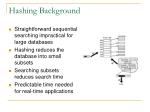Hashing Background
• Straightforward sequential searching impractical for large databases
• Hashing reduces the database into small subsets
• Searching subsets reduces search time
• Predictable time needed for real-time applications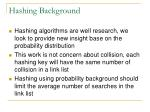Hashing Background
• Hashing algorithms are well research, we look to provide new insight base on the probability distribution
• This work is not concern about collision, each hashing key will have the same number of collision in a link list
• Hashing using probability background should limit the average number of searches in the link list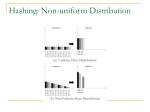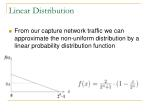Linear Distribution
• From our capture network traffic we can approximate the non-uniform distribution by a linear probability distribution function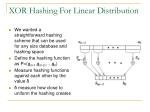XOR Hashing For Linear Distribution
• We wanted a straightforward hashing scheme that can be used for any size database and hashing space
• Define the hashing function as P=(gm-1,gm-2,…,g0)
• Measure hashing functions against each other by the value δ
• δ measure how close to uniform the hashing creates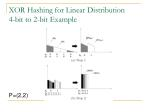XOR Hashing Observation
• Observations:
• gi > 1: leads to equal partitioning
• gi = 1: leads to unequal partitioning
• δ: difference between highest hash distribution density and mean
• To find δ: we need to determine highest final hash distribution densityOptimal XOR Hashing for Linear Distribution
• Hashing consists of m steps (from step m-1 to step 0)
• pi : highest density value after step i
• Derive pi from pi+1 at step i
• pm = A = 1/2n (original mean before hashing)
• δ = p0 – 1/2mδ vs. P for Linear Distribution
• Optimal solution comes from all groups XORing more than 1 bitSimulation
• Goal: Demonstrate that lower δ leads to better search performance
• Hashing: map from 2n to 2m
• Each simulation performs 2m hash lookupsSimulation
• Three performance measurements
• Number of Empty Bins (NEB)
• Average maximum Search Length (ASL)
• Maximum Search Length (MSL)Simulation
• Improvement from best δ over worst δ
• NEB: 18%
• ASL: 12%
• MSL: 17%Future Work
• Find optimal XOR hashing for exponential distribution and partial linear distribution
• Look more in depth to see if what applications exhibit linear distribution
• Find performance gain of using this hashing scheme in an intrusion detection systemConclusion
• Network applications demonstrate non-uniform distribution making known search techniques less than optimal
• Linear distribution can benefit from the XOR folding property
• Optimal XOR grouping can be easily identified to minimize error in hashing distribution
• Theory in linear case can be applied to other non-uniform distributions